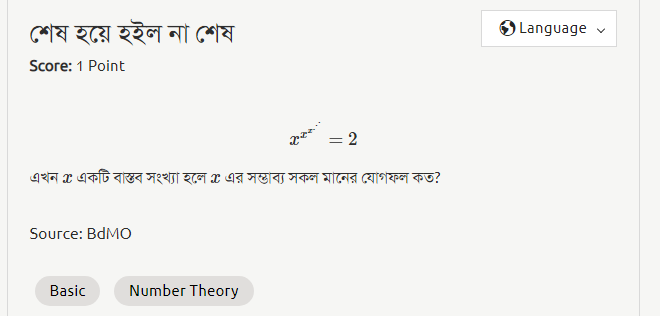# How to give Square root of 2 as an answer in the website?

Specifically for the question শেষ হয়ে হইল না শেষ

You could try using the square root symbol on your android keyboard, or write sqrt(2) or write the square root of 2 up to 3 decimal places. One of them should work.

but if your answer is something like 5\sqrt{2} then you must express this in decimal and write up to three decimal places.

1 Like

tried all of them and none workedThe answer is not √2. Try once more
By the way Can we write an irrational number as a power of any number?

^nP_r=n^r

\fbox{Answer is the value of "r".}